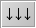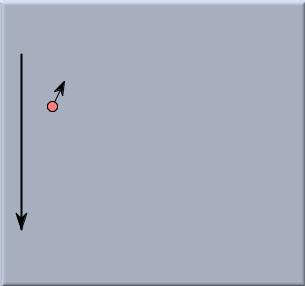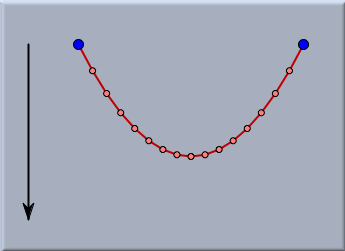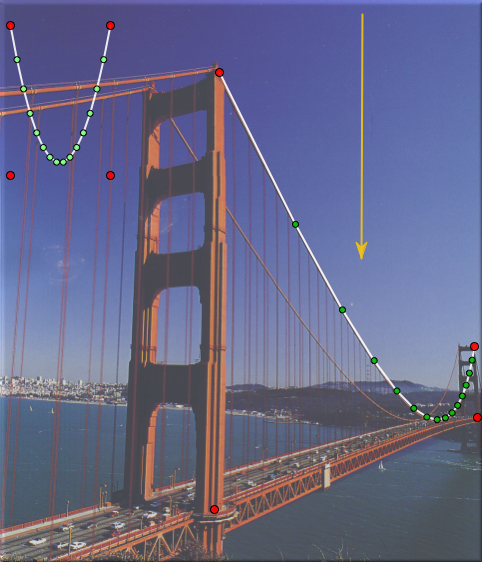# Gravitybacklinks... Bouncer CindyLab Environment Free Mass Geometry and CindyLab Rubber Band Sun SunJ Table of Contents The Elements of CindyLab English 日本語

## GravityGravity is a constant force that acts on all masses. The gravity mode in Cinderella is designed to model the gravitational force at the earth's surface, that is, a constant force field that acts on an object with nonzero mass. The force on a mass-object caused by gravity is dependent on two parameters: gravity's strength and the value of the mass of the object (you can consider this as the weight of the object, though technically, mass and weight are two different concepts). The exact relation between these three magnitudes is described by the following equation:

 force = gravityconstant ∗ mass

Gravity also has a direction toward which a mass-object is drawn. In Cinderella you create a gravitational force by a press/drag/release cycle similar to that for creating a line in Add a Line mode. The gravitational force field is represented by a black arrow that points in the direction of the force. The longer the arrow, the stronger the gravitational force.

The following pictures demonstrate how gravity acts on a mass with nonzero initial Velocity. The first picture shows the situation before the simulation is started. The second picture shows a trace of the mass-object during the simulation: a ballistic parabola.A mass-object with nonzero initial velocity before start Trace of the mass-object under the influence of gravity

As a second example we consider the action of gravity on a string of masses connected by Rubber Bands, whose endpoints are pinned to fixed positions in the drawing. Without a change in the default settings of CindyLab, the system would oscillate wildly. However, if we add some friction to the Environment, the system will enter a state of equilibrium after some time. In this equilibrium situation the chain of masses forms a perfect parabola.A chain of mass-objects under the influence of gravity

The picture below shows an even more sophisticated example. Here a background image of the Golden Gate Bridge has been loaded. The upper left part of the picture shows a physics simulation of a rubber-band chain under the influence of gravity. A Projective Transformation has been used to map the simulated situation to a rectangular frame on the Golden Gate Bridge. Adjusting the gravitational force to the correct value shows that this situation exactly resembles the situation on the supporting cables of the bridge.An analysis of the Golden Gate Bridge

### Inspecting Gravity

A CindyLab gravitational field is equipped with a built-in scaling factor that is set to a relatively small value. The actual value of the gravityconstant is calculated as this factor times the length of the gravity arrow in the drawing.The gravity inspector

### Gravity and CindyScript

Like any CindyLab object, a gravitational force provides several fields that can be read and very often set by CindyScript. The following list shows the accessible fields for gravity:

 Name Writeable Type Purpose strength yes real a handle to the scaling factor potential no real the overall potential energy of masses in the gravitational field, defined only up to an additive constant that depends on the choice of coordinates pe no real the overall potential energy of mass objects in the gravitational field, defined only up to an additive constant that depends on the choice of coordinates simulate yes bool turn on/off simulation for the gravitational field